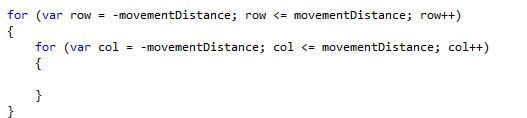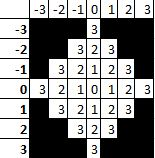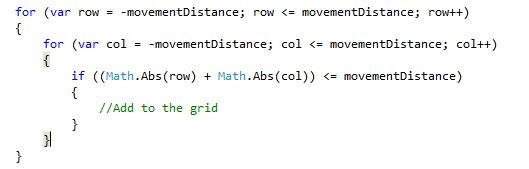Movement grid

How to create a movment grid using c# (XNA / WP7) at runtime.

Posted by on - Intermediate Mapping/Technical

I have got the movement grid calculating on the fly from the current MP of the selected character.

In order to do this I had to work out a simple function, to calculate what cells should be rendered.

I wanted the grid to be centered on the characters position therefore I knew I would have to negativly and positively from the orgin.

For this example lets take it that the character is at 10,10 and the movement points were 3.

Using a foreach loop you can easily iterate through and create a grid.Now that the loop has been created you need a way of checking that the distance is within range.

The image below shows x + y cell values when the - and + are dropped.In c# there is a built in function called ABS (Math.Abs) that allows you to get the absolute value from a number allowing -3 to be the same as +3.

This allows for the following loop:Here is the working code from my game: